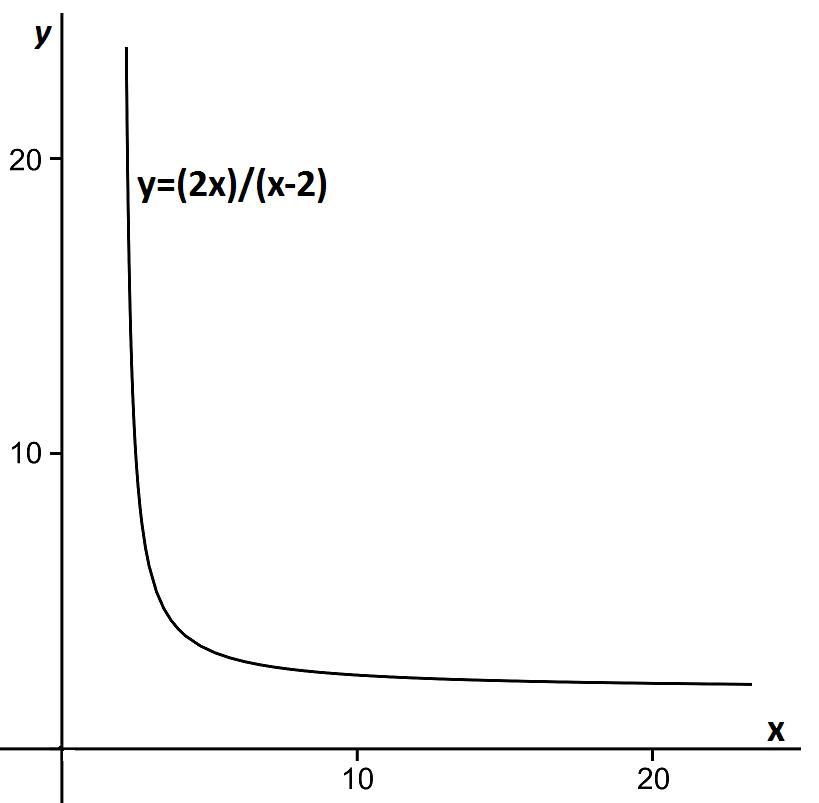#### You may also like### Framed

Seven small rectangular pictures have one inch wide frames. The frames are removed and the pictures are fitted together like a jigsaw to make a rectangle of length 12 inches. Find the dimensions of the pictures.### Tilted Squares

It's easy to work out the areas of most squares that we meet, but what if they were tilted?### Four or Five

The diagram shows a large rectangle composed of 9 smaller rectangles. If each of these rectangles has integer sides, what could the area of the large rectangle be?

# Can They Be Equal?

##### Age 11 to 14Challenge Level

We had lots of solutions to this problem, so well done to everyone who submitted an answer!

David, Noah, Felix, Tom, Amy and Laura from Bristol Grammar School worked on this problem together. David says:

We found that the 6 by 3 rectangle works, because 6+3+6+3=18 and 6x3=18, so this has equal area and perimeter.

Laura and Amy say:

We have been working systematically to list all the possible rectangles, e.g. 1x1 1x2 1x3 1x4 ... and deciding whether there are cases with 1, 2 or 3

2x1 2x2 2x3 2x4...
3x1 3x2 3x3 3x4...

We noticed a diagonal pattern for where the perimeter becomes less than the area.
We are still working on a solution!

Well done to Gourav from India, Radha from Stanhope, Kirstey from da Vinci College and Caitlin from Marshfield Primary who all correctly found rectangles with the same area and perimeter, such as 4x4 and 3x6.

Hannah from Leicester Girls High made some good notes on even and odd numbers:

I realised that at least one of the length or the width of the rectangle has to be even. The perimeter will always be even, because the length is multiplied by 2, making it even, and is added to the width which has been multiplied by 2, also making it even. But if both the length and the width are odd, then the area will be odd, meaning that it is impossible for the perimeter to be the same as the area.

Click here to see how Dexter from Wilson's School used diagrams to understand different rectangles and how he also used some algebra to find examples of rectangles where the perimeter is equal to the area.

Lucy from Belgium noticed visually what was happening to rectangles with whole number side lengths:

Each square along the edges accounts for one unit of perimeter, except for the four corner squares. They account for two units of perimeter but only one unit of area. This means the perimeter is 4 more than the area and there will have to be some squares in the middle that will only be counted for the area and not the perimeter.

Bhavik from Queen Elizabeth's School for Boys also considered rectangles with whole number side lengths, and came to the same conclusion. Click here to see his very clear explanation of why there can only be two such rectangles where the area is equal to the perimeter.

Nathan from Rushmore Primary made an attempt at using algebra, which was continued by Vicki from Farnborough Hill, Eliza and Jacqueline from Chevalier College, Australia and Tom. Vicki noted that for a rectangle x by y, the area is equal to the perimeter if: \begin{align*} xy &= 2x+2y \\ xy-2y &= 2x \\ y(x-2) &= 2x\\ y &= \frac {2x}{x-2} \end{align*}

Niharika then looked at the possible values of x and y from this equation: \begin{align*} y &= \frac {2x}{x-2} \text{ and } x ,y> 0 \\ \Rightarrow x-2 &> 0 \\ x&> 2 \end{align*} There are an infinite number of these rectangles.

Substituting in different values of x and y and checking the answers are correct is a good problem solving skill - well done to Krystof and Mimas who did this. Also Shashank from India drew a graph of the possible x and y values:Esther commented that:

Although you can always put a number into the formula I used, $2x+2y=xy$, the end result is not always an integer.
In fact, the only rectangles like this with side lengths integers are a 3x6 rectangle and a 4x4 square.
Any other numbers substituted into the formula as x will give a decimal output for y.
For example, if you put in 5 as x, your value for y would be 10/3.

Well done to Miss Gerrard's 2BE Class from Perth High School, who noticed some interesting connections between area and perimeter:

We all found 2 rectangles that worked fairly quickly. They were the square with sides of 4 (A=P=16) and the rectangle with sides 3 and 6 (A=P=18).

Then we decided that we would try using decimals to see if we could find any more as we were getting stuck. One of us found out that a rectangle of sides 10 and 2.5 worked as it gave us an area and perimeter of 25.

After we found this rectangle someone else in the class managed to spot a pattern linking the numbers. Start at the square with sides 4 by 4.
Then look at the rectangle with side length of 3 and width of 6.
The difference between the lengths 3 and 4 is 1, the difference between the widths 4 and 6 is 2.
To get from 3 to the next length you halve the difference between 4 and 3 and subtract this from 3, to get 2.5.
To get from 6 to the next width you double the difference between 4 and 6 and add this to 6, to get 10.
We found out that if you continue halving the difference in lengths and subtracting this and doubling the width and adding it on you can find many more rectangles with equal perimeter and area:
$4$ by $4$
$3$ by $6$
$2\frac{1}{2}$ by $10$
$2\frac{1}{4}$ by $18$
$2\frac{1}{8}$ by $34$
...

Well done to you all.